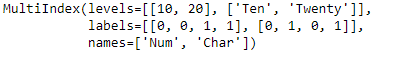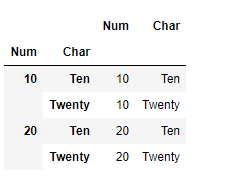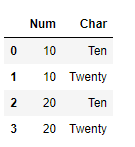# Python | Pandas MultiIndex.to_frame()

## Python | Pandas MultiIndex.to_frame()

Python is a great language for doing data analysis, primarily because of the fantastic ecosystem of data-centric python packages. Pandas is one of those packages and makes importing and analyzing data much easier.

Pandas` MultiIndex.to_frame()` function create a DataFrame with the levels of the MultiIndex as columns.

Syntax: MultiIndex.to_frame(index=True)

Parameters :
index : Set the index of the returned DataFrame as the original MultiIndex.

Returns : DataFrame : a DataFrame containing the original MultiIndex data.

Example #1: Use `MultiIndex.to_frame()` function to construct a dataframe using the MultiIndex levels as the column and index.

 `# importing pandas as pd` `import` `pandas as pd` ` ` `# Create the MultiIndex` `midx ``=` `pd.MultiIndex.from_tuples([(``10``, ``'Ten'``), (``10``, ``'Twenty'``), ` `                                  ``(``20``, ``'Ten'``), (``20``, ``'Twenty'``)], ` `                                        ``names ``=``[``'Num'``, ``'Char'``])` ` ` `# Print the MultiIndex` `print``(midx)`

Output :Now let’s construct the dataframe from the MultiIndex.

 `# Construct the DataFrame` `midx.to_frame(index ``=` `True``)`

Output :As we can see in the output, the function has constructed the Dataframe using the MultiIndex. Notice the index of the dataframe is constructed using the levels of the MultiIndex.

Example #2: Use `MultiIndex.to_frame()` function to construct a DataFrame using the MultiIndex. Do not use the MultiIndex levels to construct the index of the Dataframe.

 `# importing pandas as pd` `import` `pandas as pd` ` ` `# Create the MultiIndex` `midx ``=` `pd.MultiIndex.from_tuples([(``10``, ``'Ten'``), (``10``, ``'Twenty'``),  ` `                                  ``(``20``, ``'Ten'``), (``20``, ``'Twenty'``)], ` `                                        ``names ``=``[``'Num'``, ``'Char'``])` ` ` `# Print the MultiIndex` `print``(midx)`

Output :Now let’s create a dataframe using the midx MultiIndex.

 `# Create Dataframe with new index values.` `midx.to_frame(index ``=` `False``)`

Output :As we can see in the output, the function has returned a DataFrame having different index value.

Last Updated on October 23, 2021 by admin

## Pandas dataframe.drop_duplicates()Pandas dataframe.drop_duplicates()

Python | Pandas dataframe.drop_duplicates() Python is a great language for doing data analysis, primarily because

## How to Move a Column to First Position in Pandas DataFrame?How to Move a Column to First Position in Pandas DataFrame?

How to Move a Column to First Position in Pandas DataFrame? The basic idea to

## Set Pandas dataframe background Color and font color in PythonSet Pandas dataframe background Color and font color in Python

Set Pandas dataframe background Color and font color in Python As we know, the basic

## How to create multiple CSV files from existing CSV file using Pandas ?How to create multiple CSV files from existing CSV file using Pandas ?

How to create multiple CSV files from existing CSV file using Pandas ? In this

## Extract week number from date in Pandas-PythonExtract week number from date in Pandas-Python

Extract week number from date in Pandas-Python Many times, when working with some data containing

## Pandas Series.str.zfill()Pandas Series.str.zfill()

Python | Pandas Series.str.zfill() Python is a great language for doing data analysis, primarily because

## Replace Negative Number by Zeros in Pandas DataFrameReplace Negative Number by Zeros in Pandas DataFrame

Replace Negative Number by Zeros in Pandas DataFrame In this article, Let’s discuss how to